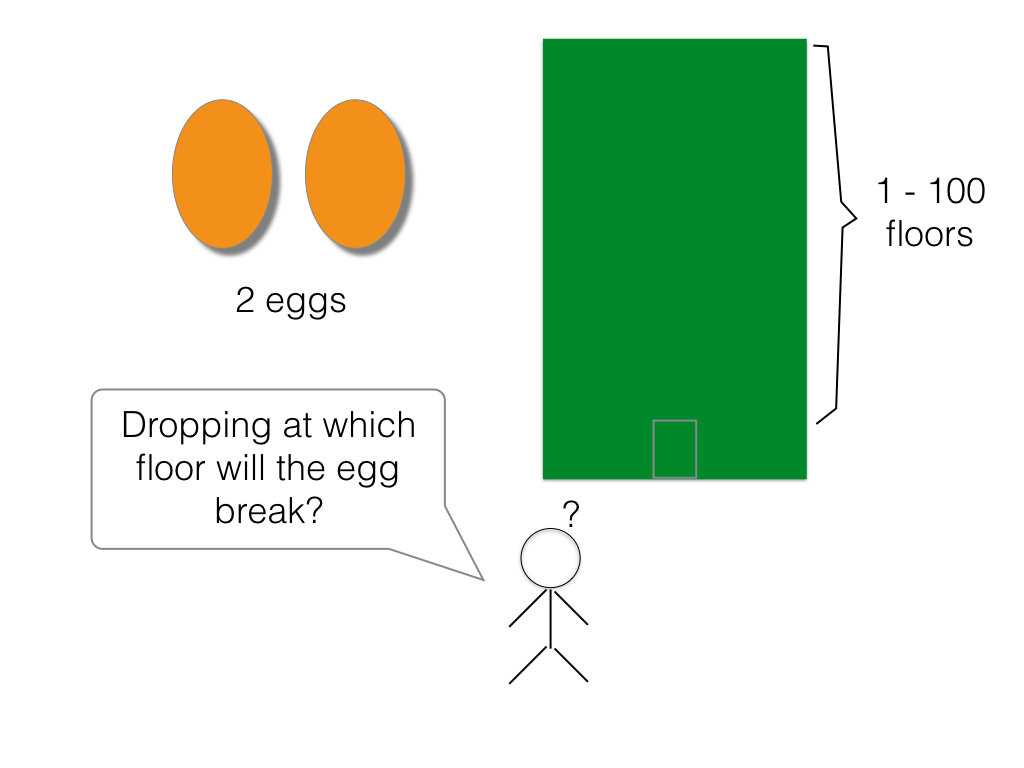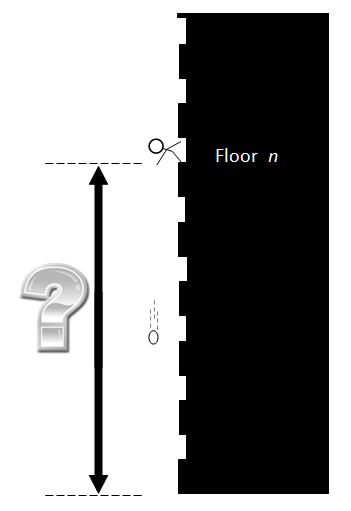Juice is good for health.Default error msg

New to Study24x7 ?

# 2 Eggs and 100 Floors Puzzle

Updated on 16 June 2023Data Structure
Updated on 16 June 2023# Puzzle Definition

You are given two eggs, and access to a 100-storey building.Both eggs are identical. The aim is to find out the highest floor from which an egg will not break when dropped out of a window from that floor. If an egg is dropped and does not break, it is undamaged and can be dropped again. However, once an egg is broken, that’s it for that egg.

If an egg breaks when dropped from floor n, then it would also have broken from any floor above that. If an egg survives a fall, then it will survive any fall shorter than that.

## The question is:

What strategy should you adopt to minimise the number egg drops it takes to find the solution?. (And what is the worst case for the number of drops it will take?)

There are no tricks, gotchas or other devious ruses. Don’t rat-hole with issues related to terminal velocity, potential energy or wind resistance. This is a maths puzzle plain and simple.

Think about the puzzle for a few minutes, and then read on.## Solution :

An egg could break at any time.So we have to find that how many floors can we explore with a single egg?

Since we can’t afford to lose our only egg, we’re forced to start at the ground floor. If the egg breaks, then we’ve found the right floor. Otherwise, we get to keep our egg, and we can try again with the next floor. Continuing on like this, the distance we can explore with 1 egg .

Let’s break out some algebra.

Imagine we drop our first egg from floor n, if it breaks, we can step through the previous (n-1) floors one-by-one.

If it doesn’t break, rather than jumping up another n floors, instead we should step up just (n-1) floors (because we have one less drop available if we have to switch to one-by-one floors), so the next floor we should try is floor n + (n-1)

Similarly, if this drop does not break, we next need to jump up to floor n + (n-1) + (n-2), then floor n + (n-1) + (n-2) + (n-3) …

We keep reducing the step by one each time we jump up, until that step-up is just one floor, and get the following equation for a 100-floor building:

## n + (n-1) + (n-2) + (n-3) + (n-4) + … + 1 >= 100

This summation, as many will recognise, is the formula for triangular numbers (which kind of makes sense, since we’re reducing the step by one each drop we make) and can be simplified to:

## n (n+1) / 2 >= 100

This is a quadratic equation, with the positive root of 13.651 (Which we have to round up to 14).

## Explanation:

Our first drop should be from floor 14, if egg survives we step up 13 floors to floor 27, then up 12 floors to 39 …

The optimal strategy is to work our way through the table until the first egg breaks, then back up to one floor higher than the line above and then proceed floor-by-floor until we find the exact solution.

This complete table is shown in here on the left.

The maximum of 14 drops is a combination first egg drops (made in steps), and second egg drops (made in ones). For every drop we take hopping up the tower, we reduce the worst-case number of single drops we’d have to take so that no solution is an outlier.

## #12 100

Write a comment...Trending ArticlesBy CBSE CLASS 8By CBSE CLASS 10By Edubabble LLPBy CBSE CLASS 7
Related PostsServicesNeed Some Help!Connect